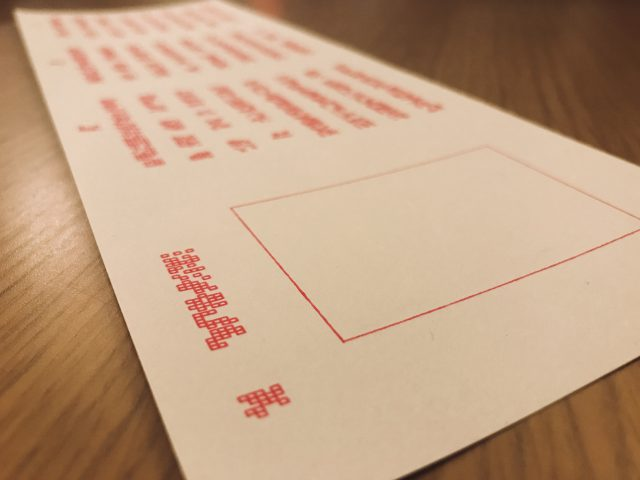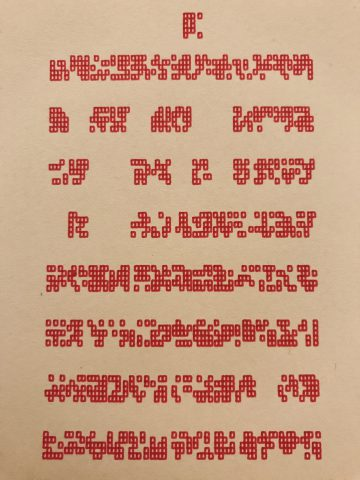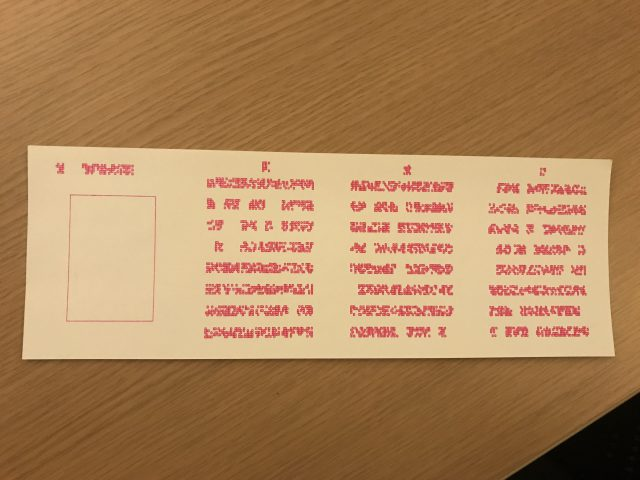# zbeok-Asemic

In making my asemic script, I looked into existing methods of inscribing information. At first I thought of ciphers, but I moved onto thinking about binary encoding of information. It reminded me of Braille, so I jumbled the ideas together to bring a dense informational paragraph script together. It is designed with the idea of space-efficient encoding of information, like binary code, but not too evocative of technology. I’ve printed in pamphlet form so that it further represents this dense chunk of information. The plotter adds inaccuracies that is more human than machine. What’s different about using a plotter device than a screen?

DOCUMENTATION

Sketches:PDF screenshot:Plotting Process:

Video```// see https://processing.org/reference/libraries/pdf/index.html
import processing.pdf.*;
boolean bRecordingPDF;
int pdfOutputCount = 0;

int gridr = 15;
int gridc = 13;
int txtw = 1200/4*3/4;
int txth = 900/2*3/4;

void setup() {
size(1200, 900);
bRecordingPDF = true;
noFill();
strokeWeight(1.5);
}

void keyPressed() {
// When you press a key, it will initiate a PDF export
bRecordingPDF = true;
}

void draw() {
if (bRecordingPDF) {
background(255); // this should come BEFORE beginRecord()
beginRecord(PDF, "myName_" + pdfOutputCount + ".pdf");
int dx = (width/4-txtw)/2;
int dy = (height/2-txth)/2;

rect(dx+txtw*1/8,dy+txth*1/8,txtw*3/4,txth*3/4);
writeIt(gridc*3/4,1,dx,dy*5/8);
for (int u=1;u<4;u++) {
//fill(255,u*70,u*70);
int ndx = dx+(2*dx)*(u)+txtw*u;
//rect(width/4*u,0,width/4,height/2);
writeIt(gridc,gridr,ndx,dy);
writeIt(1,1,ndx+txtw/2,dy*3/8);
}
int ndy = (height/2-txth)/2+height/2;
for (int b=1;b<4;b++) {
//fill(255,b*70,b*70);
int ndx = dx+(2*dx)*(b)+txtw*b;
//rect(width/4*b,height/2,width/4,height/2);
writeIt(gridc,gridr,ndx,ndy);
writeIt(1,1,ndx+txtw/2,ndy+txth+dy*1/8);
}
endRecord();
bRecordingPDF = false;
pdfOutputCount++;
}
}

void writeIt(int cols, int rows, int dx, int dy) {
int gridw = txtw/gridc;
int gridh = txth/gridr;
print(gridh,txth,"\n");
for (int i=0;i.1) {
int x1 = j*gridw;
int x2 = (j+1)*gridw;
int y1 = i*gridh;
int y2 = (i+1)*gridh;
braille(x1 +dx,x2+dx,y1+dy,y2+dy);
}
}
}
}
}

void braille(int x1,int x2,int y1,int y2) {
int rows = 5;
int cols = 5;
int w = (x2-x1)/cols;
int h = (y2-y1)/rows;
stroke(0);
for (int i=0;i.5) {
//strokeWeight(1);
rect(x1+i*w,y1+j*h,w,h);
strokeWeight(1.5);
//point((2*x1+(2*j+1)*w)/2,(2*y1+(2*i+1)*h)/2);
//drawUnit(x1+i*w,x1+(i+1)*w,y1+j*h,y1+(j+1)*h);
}
}
}
}
```